JMSLTM Numerical Library 7.2.0
com.imsl.math

## Class BoundedLeastSquares

• All Implemented Interfaces:
Serializable, Cloneable

```public class BoundedLeastSquares
extends Object
implements Serializable, Cloneable```
Solves a nonlinear least-squares problem subject to bounds on the variables using a modified Levenberg-Marquardt algorithm.

Class `BoundedLeastSquares` uses a modified Levenberg-Marquardt method and an active set strategy to solve nonlinear least-squares problems subject to simple bounds on the variables. The problem is stated as follows: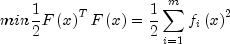subject to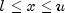where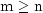,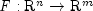, and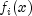is the i-th component function of F(x). From a given starting point, an active set `IA`, which contains the indices of the variables at their bounds, is built. A variable is called a "free variable" if it is not in the active set. The routine then computes the search direction for the free variables according to the formula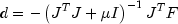where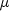is the Levenberg-Marquardt parameter, F = F(x), and J is the Jacobian with respect to the free variables. The search direction for the variables in `IA` is set to zero. The trust region approach discussed by Dennis and Schnabel (1983) is used to find the new point. Finally, the optimality conditions are checked. The conditions are: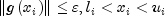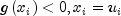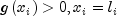whereis a gradient tolerance. This process is repeated until the optimality criterion is achieved.

The active set is changed only when a free variable hits its bounds during an iteration or the optimality condition is met for the free variables but not for all variables in `IA`, the active set. In the latter case, a variable that violates the optimality condition will be dropped out of `IA`. For more details on the Levenberg-Marquardt method, see Levenberg (1944) or Marquardt (1963). For more detail on the active set strategy, see Gill and Murray (1976).

Example 1, Example 2, Serialized Form
• ### Nested Class Summary

Nested Classes
Modifier and Type Class and Description
`static class ` `BoundedLeastSquares.FalseConvergenceException`
False convergence - The iterates appear to be converging to a noncritical point.
`static interface ` `BoundedLeastSquares.Function`
Public interface for the user-supplied function to evaluate the function that defines the least-squares problem.
`static interface ` `BoundedLeastSquares.Jacobian`
Public interface for the user-supplied function to compute the Jacobian.
• ### Constructor Summary

Constructors
Constructor and Description
```BoundedLeastSquares(BoundedLeastSquares.Function function, int mFunctions, int nVariables, int boundType, double[] lowerBound, double[] upperBound)```
Constructor for `BoundedLeastSquares`.
• ### Method Summary

Methods
Modifier and Type Method and Description
`double[][]` `getJacobian()`
Returns the Jacobian at the approximate solution.
`int` `getNumberOfThreads()`
Returns the number of `java.lang.Thread` instances used for parallel processing.
`double[]` `getResiduals()`
Returns the residuals at the approximate solution.
`double[]` `getSolution()`
Returns the solution.
`void` `setAbsoluteFcnTol(double absoluteFcnTol)`
Sets the absolute function tolerance.
`void` `setDiagonalScalingMatrix(double[] diagonalScalingMatrix)`
Sets the diagonal scaling matrix for the functions.
`void` `setGoodDigit(int goodDigit)`
Sets the number of good digits in the function.
`void` `setGradientTol(double gradientTol)`
`void` `setGuess(double[] guess)`
Sets the initial guess of the solution.
`void` `setInternalScale()`
Sets the internal variable scaling option.
`void` `setJacobian(BoundedLeastSquares.Jacobian jacobian)`
Sets the Jacobian.
`void` `setMaximumFunctionEvals(int evaluations)`
Sets the maximum number of function evaluations.
`void` `setMaximumIteration(int iterations)`
Sets the maximum number of iterations.
`void` `setMaximumJacobianEvals(int evaluations)`
Sets the maximum number of Jacobian evaluations.
`void` `setMaximumStepSize(double stepSize)`
Sets the maximum allowable step size.
`void` `setNumberOfThreads(int numberOfThreads)`
Sets the number of `java.lang.Thread` instances to be used for parallel processing.
`void` `setRelativeFcnTol(double relativeFcnTol)`
Sets the relative function tolerance.
`void` `setScaledStepTol(double scaledStepTol)`
Sets the scaled step tolerance.
`void` `setScalingVector(double[] scalingVector)`
Sets the scaling vector for the variables.
`void` `setTrustRegion(double trustRegion)`
Sets the size of initial trust region radius.
`void` `solve()`
Solves a nonlinear least-squares problem subject to bounds on the variables using a modified Levenberg-Marquardt algorithm.
• ### Methods inherited from class java.lang.Object

`clone, equals, finalize, getClass, hashCode, notify, notifyAll, toString, wait, wait, wait`
• ### Constructor Detail

• #### BoundedLeastSquares

```public BoundedLeastSquares(BoundedLeastSquares.Function function,
int mFunctions,
int nVariables,
int boundType,
double[] lowerBound,
double[] upperBound)```
Constructor for `BoundedLeastSquares`.
Parameters:
`function` - a `Function` object, user-supplied function to evaluate the function
`mFunctions` - an `int` scalar containing the number of functions
`nVariables` - an `int` scalar containing the number of variables
`boundType` - an `int` scalar containing the types of bounds on the variable  boundType Action 0 User will supply all the bounds. 1 All variables are nonnegative. 2 All variables are nonpositive. 3 User supplies only the bounds on first variable, all other variables will have the same bounds.
`lowerBound` - a `double` array containing the lower bounds on the variables
`upperBound` - a `double` array containing the upper bounds on the variables
Throws:
`IllegalArgumentException` - is thrown if the dimensions of `mFunctions`, `nVariables`, `boundType`, `lowerBound.length` and `upperBound.length` are not consistent
• ### Method Detail

• #### getJacobian

`public double[][] getJacobian()`
Returns the Jacobian at the approximate solution.
Returns:
a `mFunctions x nVariables double` matrix containing the Jacobian at the approximate solution

`public int getNumberOfThreads()`
Returns the number of `java.lang.Thread` instances used for parallel processing.
Returns:
an `int` containing the number of `java.lang.Thread` instances used for parallel processing.
• #### getResiduals

`public double[] getResiduals()`
Returns the residuals at the approximate solution.
Returns:
a `double` array containing the residuals at the approximate solution
• #### getSolution

`public double[] getSolution()`
Returns the solution.
Returns:
a `double` array containing the computed solution
• #### setAbsoluteFcnTol

`public void setAbsoluteFcnTol(double absoluteFcnTol)`
Sets the absolute function tolerance. If this member function is not called, a value of Math.max(1.0e-10, Math.pow(2.2204460492503131e-16, 2.0/3.0)), is used.
Parameters:
`absoluteFcnTol` - a `double` scalar containing the absolute function tolerance
• #### setDiagonalScalingMatrix

`public void setDiagonalScalingMatrix(double[] diagonalScalingMatrix)`
Sets the diagonal scaling matrix for the functions. The i-th component of the array is a positive scalar specifying the reciprocal magnitude of the i-th component function of the problem. If this member function is not called, an initial scaling of 1.0 is used.
Parameters:
`diagonalScalingMatrix` - a `double` array containing the diagonal scaling for the functions
• #### setGoodDigit

`public void setGoodDigit(int goodDigit)`
Sets the number of good digits in the function. If this member function is not called, a value of (int)(-Sfun.log10(2.2204460492503131e-16) + 0.1e0) is used.
Parameters:
`goodDigit` - an `int` scalar containing the number of good digits

`public void setGradientTol(double gradientTol)`
Sets the scaled gradient tolerance. If this member function is not called, a value of Math.pow(2.2204460492503131e-16, 1.0e0/3.0e0) is used.
Parameters:
`gradientTol` - a `double` scalar containing the scaled gradient tolerance
• #### setGuess

`public void setGuess(double[] guess)`
Sets the initial guess of the solution. If this member function is not called, an initial scaling of 1.0 is used.
Parameters:
`guess` - a `double` array containing an initial guess
• #### setInternalScale

`public void setInternalScale()`
Sets the internal variable scaling option. With this option, scaling for the variables is set internally.
• #### setJacobian

`public void setJacobian(BoundedLeastSquares.Jacobian jacobian)`
Sets the Jacobian.
Parameters:
`jacobian` - a `Jacobian` object to compute the Jacobian.
• #### setMaximumFunctionEvals

`public void setMaximumFunctionEvals(int evaluations)`
Sets the maximum number of function evaluations. If this member function is not called, a value of 400 is used.
Parameters:
`evaluations` - an `int` scalar containing the maximum number of function evaluations
• #### setMaximumIteration

`public void setMaximumIteration(int iterations)`
Sets the maximum number of iterations. If this member function is not called, a value of 100 is used.
Parameters:
`iterations` - an `int` scalar containing the maximum number of iterations
• #### setMaximumJacobianEvals

`public void setMaximumJacobianEvals(int evaluations)`
Sets the maximum number of Jacobian evaluations. If this member function is not called, a value of 400 is used.
Parameters:
`evaluations` - an `int` scalar containing the maximum number of Jacobian evaluations
• #### setMaximumStepSize

`public void setMaximumStepSize(double stepSize)`
Sets the maximum allowable step size.
Parameters:
`stepSize` - a `double` scalar containing the maximum allowable step size

`public void setNumberOfThreads(int numberOfThreads)`
Sets the number of `java.lang.Thread` instances to be used for parallel processing.
Parameters:
`numberOfThreads` - an `int` specifying the number of `java.lang.Thread` instances to be used for parallel processing. If `numberOfThreads` is greater than 1, then interface `Function.compute` is evaluated in parallel and `Function.compute` must be thread-safe. Otherwise, unexpected behavior can occur.

Default: `numberOfThreads` = 1.

• #### setRelativeFcnTol

`public void setRelativeFcnTol(double relativeFcnTol)`
Sets the relative function tolerance. If this member function is not called, a value of Math.pow(2.2204460492503131e-16, 2.0e0/3.0e0) is used.
Parameters:
`relativeFcnTol` - a `double` scalar containing the relative function tolerance
• #### setScaledStepTol

`public void setScaledStepTol(double scaledStepTol)`
Sets the scaled step tolerance. If this member function is not called, a value of Math.max(1.0e-10, Math.pow(2.2204460492503131e-16, 2.0e0/3.0e0) is used.
Parameters:
`scaledStepTol` - a `double` scalar containing the scaled step tolerance
• #### setScalingVector

`public void setScalingVector(double[] scalingVector)`
Sets the scaling vector for the variables. If this member function is not called, an initial scaling of 1.0 is used.
Parameters:
`scalingVector` - a `double` array containing the scaling vector for the variables
• #### setTrustRegion

`public void setTrustRegion(double trustRegion)`
Sets the size of initial trust region radius. If this member function is not called, the value is based on the initial scaled Cauchy step.
Parameters:
`trustRegion` - a `double` scalar containing the initial trust region radius
• #### solve

```public final void solve()
throws BoundedLeastSquares.FalseConvergenceException```
Solves a nonlinear least-squares problem subject to bounds on the variables using a modified Levenberg-Marquardt algorithm.
Throws:
`BoundedLeastSquares.FalseConvergenceException` - is thrown when the iterates appear to be converging to a noncritical point.
JMSLTM Numerical Library 7.2.0